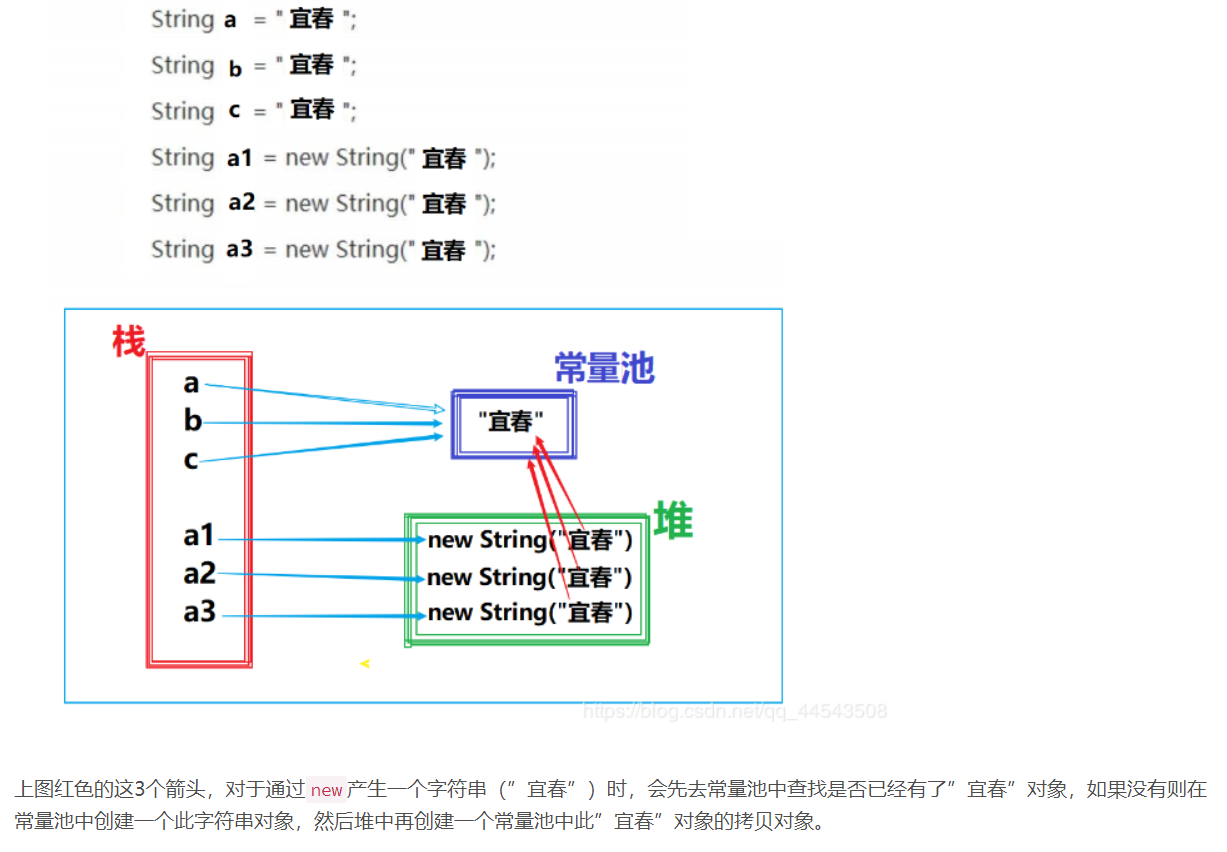# 听说你还搞不定java中的==和equals？

## 1、原生的equals()方法本身与 “ == ”没有任何区别！

  /*
* @param   obj   the reference object with which to compare.
* @return  {@code true} if this object is the same as the obj
*          argument; {@code false} otherwise.
* @see     #hashCode()
* @see     java.util.HashMap
*/
public boolean equals(Object obj) {
return (this == obj);
}


• 1、基本数据类型的变量，则直接比较其存储的 “值”是否相等；

• 2、引用类型的变量，则比较的是所指向的对象的地址是否相等；

## 2、equals()方法的重写

/**
* Compares this string to the specified object.  The result is {@code
* true} if and only if the argument is not {@code null} and is a {@code
* String} object that represents the same sequence of characters as this
* object.
*
* @param  anObject
*         The object to compare this {@code String} against
*
* @return  {@code true} if the given object represents a {@code String}
*          equivalent to this string, {@code false} otherwise
*
* @see  #compareTo(String)
* @see  #equalsIgnoreCase(String)
*/
public boolean equals(Object anObject) {
if (this == anObject) {
return true;
}
if (anObject instanceof String) {
String anotherString = (String) anObject;
int n = value.length;
if (n == anotherString.value.length) {
char v1[] = value;
char v2[] = anotherString.value;
int i = 0;
while (n-- != 0) {
if (v1[i] != v2[i])
return false;
i++;
}
return true;
}
}
return false;
}


public static void main(String[] args) {
String a = "宜春";
String b = new String("宜春");
String c = b; //注意这里是引用传递，意思是c也指向b指向的内存地址

System.out.println(a == b);  //false
System.out.println(a == c);  //false
System.out.println(b == c);  //true
System.out.println(a.equals(b));  //true
System.out.println(a.equals(c));  //true
System.out.println(b.equals(c));  //true
}


【特别注意：String类型属于引用类型】

（1）a == b？意思是地址指向的是同一块地方吗？很明显不一样。

（2）a == c？意思是地址指向的是同一块地方吗？很明显不一样。

（3）b == c？意思是地址指向的是同一块地方吗？很明显内容一样，所以为true。

（4）a.equals( b )？意思是地址指向的内容一样嘛？一样。

（4）a.equals( c )？意思是地址指向的内容一样嘛？一样。

（4）b.equals( c )？意思是地址指向的内容一样嘛？一样。OK。现在能理解嘛？你你你......不用回答，我知道你理解了（理直气壮）。当然值得注意一点的是String中intern()方法，先看一段程序：

    public static void main(String[] args) {
String a = "宜春";
String b = new String("宜春");
b=b.intern();

System.out.println(a == b);  //true
System.out.println(a.equals(b));  //true
}


intern方法的意思是检查字符串池里是否存在，如果存在了那就直接返回为true。因此在这里首先a会在字符串池里面有一个，然后 b.intern()一看池子里有了，就不再新建new了，直接把b指向它。

## 3、为什么要重写equals方法？

// 重写equals方法
@Override
public boolean equals(Object obj) {
if(!(obj instanceof Student)) {
// instanceof 已经处理了obj = null的情况
return false;
}
Student stuObj = (Student) obj;
// 对象地址相等
if (this == stuObj) {
return true;
}
// 如果两个对象姓名、身份证号码、性别相等，我们认为两个对象相等
if (stuObj.name.equals(this.name) && stuObj.sex.equals(this.sex) && stuObj.IDnumber.equals(this.IDnumber)) {
return true;
} else {
return false;
}
}


## 4、重写equals方法之后要不要重写hashCode()方法？

/**
* Returns a hash code value for the object. This method is
* supported for the benefit of hash tables such as those provided by
* <p>
* The general contract of {@code hashCode} is:
* <ul>
* <li>Whenever it is invoked on the same object more than once during
*     an execution of a Java application, the {@code hashCode} method
*     must consistently return the same integer, provided no information
*     used in {@code equals} comparisons on the object is modified.
*     This integer need not remain consistent from one execution of an
*     application to another execution of the same application.
* <li>If two objects are equal according to the {@code equals(Object)}
*     method, then calling the {@code hashCode} method on each of
*     the two objects must produce the same integer result.
* <li>It is <em>not</em> required that if two objects are unequal
*     according to the {@link java.lang.Object#equals(java.lang.Object)}
*     method, then calling the {@code hashCode} method on each of the
*     two objects must produce distinct integer results.  However, the
*     programmer should be aware that producing distinct integer results
*     for unequal objects may improve the performance of hash tables.
* </ul>
* <p>
* As much as is reasonably practical, the hashCode method defined by
* class {@code Object} does return distinct integers for distinct
* objects. (This is typically implemented by converting the internal
* address of the object into an integer, but this implementation
* technique is not required by the
* Java<font size="-2"><sup>TM</sup></font> programming language.)
*
* @return  a hash code value for this object.
* @see     java.lang.Object#equals(java.lang.Object)
* @see     java.lang.System#identityHashCode
*/
public native int hashCode();


Java中的集合List的元素是有序的，元素可以重复；Set元素无序，但元素不可重复。这我们都清楚。但是你有没有想过这样一个问题：要想保证元素不重复，可两个元素是否重复应该依据什么来判断呢？

public V put(K key, V value) {
if (table == EMPTY_TABLE) {
inflateTable(threshold);
}
if (key == null)
return putForNullKey(value);
int hash = hash(key);
//这里通过哈希值定位到对象的大概存储位置
int i = indexFor(hash, table.length);
for (Entry<K,V> e = table[i]; e != null; e = e.next) {
Object k;
//if语句中，先比较hashcode，再调用equals()比较
//由于“&&”具有短路的功能，只要hashcode不同，也无需再调用equals方法
if (e.hash == hash && ((k = e.key) == key || key.equals(k))) {
V oldValue = e.value;
e.value = value;
e.recordAccess(this);
return oldValue;
}
}

modCount++;
return null;
}


Java官方建议 重写equals()就一定要重写hashCode()方法。毕竟实际开发场景中常常用到Java集合

## 5、eqauls方法和hashCode方法关系

Java对于eqauls方法和hashCode方法是这样规定的：

1、如果两个对象equals为true ，他们的hashcode一定相等。
2、如果两个对象equals为false，他们的hashcode有可能相等。
3、如果两个对象hashcode相等，equals不一定为true。
4、如果两个对象hashcode不相等，equals一定为false。posted @ 2020-04-08 20:00  宜春  阅读(...)  评论(...编辑  收藏